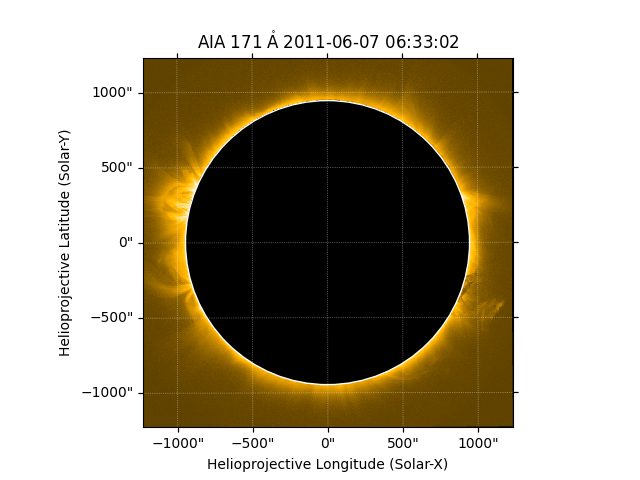# Masking out the solar disk¶

How to mask out all emission from the solar disk.

```import numpy as np
import numpy.ma as ma
import matplotlib.pyplot as plt

import sunpy.map
from sunpy.data.sample import AIA_171_IMAGE
from sunpy.map.maputils import all_coordinates_from_map
```

We start with the sample data

```aia = sunpy.map.Map(AIA_171_IMAGE)
```

A utility function gives us access to the helioprojective coordinate of each pixels. We can use that to create a new array which contains the normalized radial position for each pixel.

```hpc_coords = all_coordinates_from_map(aia)
r = np.sqrt(hpc_coords.Tx ** 2 + hpc_coords.Ty ** 2) / aia.rsun_obs
```

With this information, we create a mask where all values which are less then the solar radius are masked. We also make a slight change to the colormap so that masked values are shown as black instead of the default white.

```mask = ma.masked_less_equal(r, 1)
palette = aia.plot_settings['cmap']
palette.set_bad('black')
```

Finally we create a new map with our new mask.

```scaled_map = sunpy.map.Map(aia.data, aia.meta, mask=mask.mask)
```

Let’s plot the results using our modified colormap

```fig = plt.figure()
plt.subplot(projection=scaled_map)
scaled_map.plot(cmap=palette)
scaled_map.draw_limb()
plt.show()
```Total running time of the script: ( 0 minutes 0.839 seconds)

Gallery generated by Sphinx-Gallery